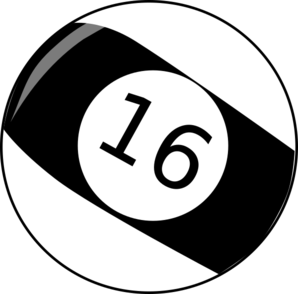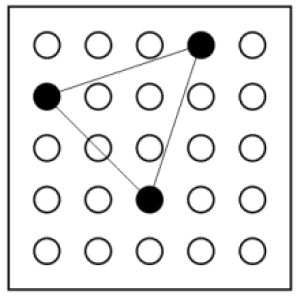## Poll

 I love math!16 votes (50%) Math is great.12 votes (37.5%) My religion is mathology.5 votes (15.62%) Women didn't speak to me until I was 30.2 votes (6.25%) Total eclipse reminder -- 04/08/202410 votes (31.25%) I steal cutlery from restaurants.3 votes (9.37%) I should just say what's on my mind.6 votes (18.75%) Who makes up these awful names for pandas?5 votes (15.62%) I like to touch my face.10 votes (31.25%) Pork chops and apple sauce.7 votes (21.87%)

32 members have voted

GialmereJoined: Nov 26, 2018
• Posts: 2055
November 27th, 2020 at 10:00:38 AM permalink
Today's puzzle is the simpler, 2d version of this week's toughie Tuesday question...From the diagram above we can see that the number of tiles on the perimeter, 16, exceeds the number of tiles on the inside, 8.

How many rectangles exist for which the number of tiles on the perimeter are equal to the number of tiles on the inside?
Have you tried 22 tonight? I said 22.
WizardJoined: Oct 14, 2009
• Posts: 23315
Thanks for this post from:November 27th, 2020 at 11:13:54 AM permalink
Quote: Gialmere

How many rectangles exist for which the number of tiles on the perimeter are equal to the number of tiles on the inside?

2

The following are the dimensions of the inner rectangle:

3x10
4x6
It's not whether you win or lose; it's whether or not you had a good bet.
ThatDonGuyJoined: Jun 22, 2011
• Posts: 4927
Thanks for this post from:November 27th, 2020 at 12:26:01 PM permalink

Let H and W be the dimensions, with H >= W
There are a total of HW squares, of which (H-2)(W-2) are interior
The number of interior = the number of exterior when HW = 2 (H-2)(W-2) = 2HW - 4H - 4W + 8
This is HW = 4H + 4W - 8
H (W - 4) = 4W - 8
H = (4W - 8) / (W - 4)
H = (4W - 16 + 8) / (W - 4)
H = 4 + 8 / (W - 4)
Since H is an integer, (W - 4) is a factor of 8
The only factors of 8 are 1, 2, 4, and 8, with resulting H values of 12, 8, 6, and 5, but the latter two have W > H
The only H values where H > W are 12 and 8; the corresponding W values are 5 and 6

12 x 5 and 8 x 6 are the only solutions

GialmereJoined: Nov 26, 2018
• Posts: 2055
Thanks for this post from:November 27th, 2020 at 8:45:18 PM permalink
Quote: Wizard

2

The following are the dimensions of the inner rectangle:

3x10
4x6

Quote: ThatDonGuy

Let H and W be the dimensions, with H >= W
There are a total of HW squares, of which (H-2)(W-2) are interior
The number of interior = the number of exterior when HW = 2 (H-2)(W-2) = 2HW - 4H - 4W + 8
This is HW = 4H + 4W - 8
H (W - 4) = 4W - 8
H = (4W - 8) / (W - 4)
H = (4W - 16 + 8) / (W - 4)
H = 4 + 8 / (W - 4)
Since H is an integer, (W - 4) is a factor of 8
The only factors of 8 are 1, 2, 4, and 8, with resulting H values of 12, 8, 6, and 5, but the latter two have W > H
The only H values where H > W are 12 and 8; the corresponding W values are 5 and 6

12 x 5 and 8 x 6 are the only solutions

Correct!
---------------------------------------

The Wi-Fi at my house went out this Thanksgiving.

There are 148 tiles in my bathroom.
Have you tried 22 tonight? I said 22.
GialmereJoined: Nov 26, 2018
• Posts: 2055
November 30th, 2020 at 8:35:38 AM permalink
I have some difficult puzzles this week so even easy Monday isn't all that easy...You go to visit your pool playing friend and shoot a few games. He's a very superstitious player and had a special 16-ball made to replace his table's 8-ball.

He also insists on racking the balls in a certain way. He arranges them so that each ball is the difference of the two balls above it. He begins to show you how to do it when his phone rings and he leaves the room.

Can you figure out how to finish racking the balls?Have you tried 22 tonight? I said 22.
ThatDonGuyJoined: Jun 22, 2011
• Posts: 4927
Thanks for this post from:November 30th, 2020 at 10:29:05 AM permalink
Quote: Gialmere

Can you figure out how to finish racking the balls?

It's not so much hard as exhaustive, as it's a bit of a brute force problem, but the solution is:
5, 14, 16, 3, 15
9, 2, 13, 12
7, 11, 1
4, 10
6

GialmereJoined: Nov 26, 2018
• Posts: 2055
November 30th, 2020 at 5:08:12 PM permalink
Quote: ThatDonGuy

It's not so much hard as exhaustive, as it's a bit of a brute force problem, but the solution is:
5, 14, 16, 3, 15
9, 2, 13, 12
7, 11, 1
4, 10
6

Correct!
--------------------------------Have you tried 22 tonight? I said 22.
GialmereJoined: Nov 26, 2018
• Posts: 2055
December 1st, 2020 at 9:04:07 AM permalink
It's toughie Tuesday...A 5 by 5 square lattice is formed by drilling holes in a piece of wood. Three pegs are placed in this lattice at random.

What is the probability that the three pegs will form a triangle?
Have you tried 22 tonight? I said 22.
charliepatrickJoined: Jun 17, 2011
• Posts: 2424
December 1st, 2020 at 9:31:11 AM permalink
Total perms =25*24*23/6 = 2208
For a triangle to have zero area the holes must be in the same line, diagonal or horizontal/vertical.
Diagonal 3-length lines - 4 of them, 1 perm = 4 combos
Diagonal 4-length lines - 4 of them, 4 perms = 16 combos
Diagonal 5-length lines - 2 of them, 10 perms = 20 combos
Vertical/Horizontal 5-length lines - 10 of them, 10 perms = 100 combos
Total = 140 combos.
So P=140/2208.
Postscript: I know this is wrong (I was on a zoom call at the time!). I agree with the comments made later in the thread.
Last edited by: charliepatrick on Dec 1, 2020
USpapergamesJoined: Jun 23, 2020